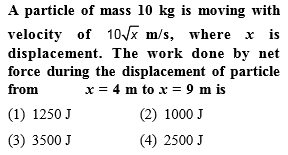NEET Questions SolvedConcept Videos :-

#1 | Work: Definition
#2 | Solved Problems

Concept Questions :-

Concept of work
Explanation is a part of a Paid Course. To view Explanation Please buy the course.

Difficulty Level:

The driver of a car travelling at velocity v suddenly see a broad wall in front of him at a distance d. He should

(1) Brake sharply

(2) Turn sharply

(3) (1) and (2) both

(4) None of the above

Concept Videos :-

#1 | Work: Definition
#2 | Solved Problems

Concept Questions :-

Concept of work
Explanation is a part of a Paid Course. To view Explanation Please buy the course.

Difficulty Level:

Work done by a frictional force is

(1) Negative

(2) Positive

(3) Zero

(4) All of the above

Concept Videos :-

#1 | Work: Definition
#2 | Solved Problems

Concept Questions :-

Concept of work
Explanation is a part of a Paid Course. To view Explanation Please buy the course.

Difficulty Level:

A block of mass 50 kg slides over a horizontal distance of 1 m. If the coefficient of friction between their surfaces is 0.2, then work done against friction is

(1) 98 J

(2) 72J

(3) 56 J

(4) 34 J

Concept Videos :-

#1 | Work: Definition
#2 | Solved Problems

Concept Questions :-

Concept of work
Explanation is a part of a Paid Course. To view Explanation Please buy the course.

Difficulty Level:

A body of mass m is moving in a circle of radius r with a constant speed v. The force on the body is $\frac{m{v}^{2}}{r}$ and is directed towards the centre. What is the work done by this force in moving the body over half the circumference of the circle

(a)       $\frac{m{v}^{2}}{\mathrm{\pi }{r}^{2}}$                   (b) Zero

(c)       $\frac{m{v}^{2}}{{r}^{2}}$                   (d)$\frac{\pi {r}^{2}}{m{v}^{2}}$

Concept Videos :-

#1 | Work: Definition
#2 | Solved Problems

Concept Questions :-

Concept of work
Explanation is a part of a Paid Course. To view Explanation Please buy the course.

Difficulty Level:

A body of mass m is moving in a circle of radius r with a constant speed v. The force on the body is $\frac{m{v}^{2}}{r}$ and is directed towards the centre. What is the work done by this force in moving the body over half the circumference of the circle

(1) $\frac{m{v}^{2}}{\pi {r}^{2}}$

(2) Zero

(3) $\frac{m{v}^{2}}{{r}^{2}}$

(4) $\frac{\pi {r}^{2}}{m{v}^{2}}$

Concept Videos :-

#1 | Work: Definition
#2 | Solved Problems

Concept Questions :-

Concept of work
Explanation is a part of a Paid Course. To view Explanation Please buy the course.

Difficulty Level:

A man pushes a wall and fails to displace it. He does

(1) Negative work

(2) Positive but not maximum work

(3) No work at all

(4) Maximum work

Concept Videos :-

#1 | Work: Definition
#2 | Solved Problems

Concept Questions :-

Concept of work
Explanation is a part of a Paid Course. To view Explanation Please buy the course.

Difficulty Level:

A body moves a distance of 10 m along a straight line under the action of a force of 5 N. If the work done is 25 joules, the angle which the force makes with the direction of motion of the body is

(1) 0°

(2) 30°

(3) 60°

(4) 90°

Concept Videos :-

#1 | Work: Definition
#2 | Solved Problems

Concept Questions :-

Concept of work
Explanation is a part of a Paid Course. To view Explanation Please buy the course.

Difficulty Level:

A force $F=\left(5\stackrel{^}{i}+3\stackrel{^}{j}\right)$ newton is applied over a particle which displaces it from its origin to the point $r=\left(2\stackrel{^}{i}-1\stackrel{^}{j}\right)$  metres. The work done on the particle is

(1) – 7 joules

(2) + 13 joules

(3) + 7 joules

(4) + 11 joules

Concept Videos :-

#1 | Work: Definition
#2 | Solved Problems

Concept Questions :-

Concept of work
Explanation is a part of a Paid Course. To view Explanation Please buy the course.

Difficulty Level:

The work done in pulling up a block of wood weighing 2 kN for a length of 10m on a smooth plane inclined at an angle of 15° with the horizontal is

(1) 4.36 kJ

(2) 5.17 kJ

(3) 8.91 kJ

(4) 9.82 kJ

Concept Videos :-

#1 | Work: Definition
#2 | Solved Problems

Concept Questions :-

Concept of work
Explanation is a part of a Paid Course. To view Explanation Please buy the course.

Difficulty Level: